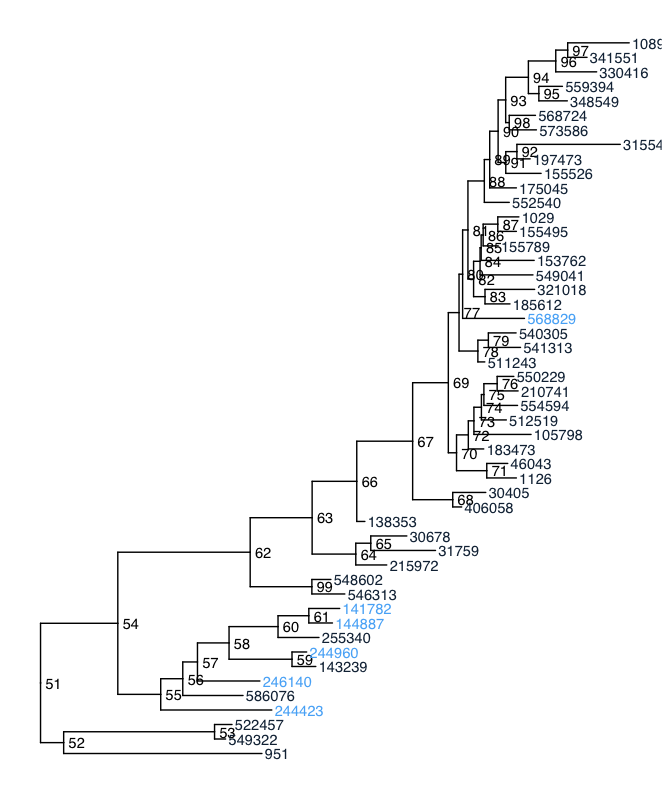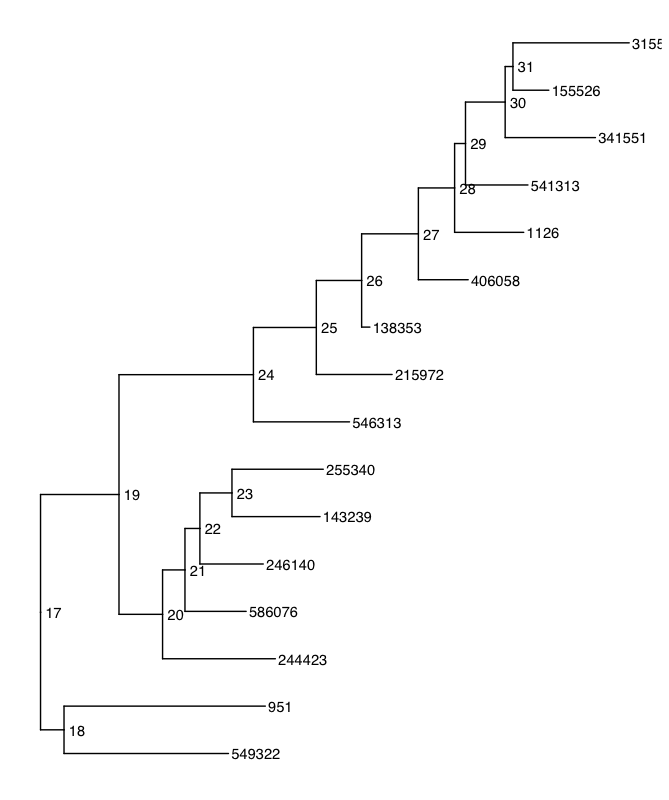tip_glom and annotations?
1
0
Entering edit mode
werdnus • 0
@werdnus-14973
Last seen 4.5 years ago

Okay, I've been banging my head against this wall for too long, and it can't just be me having this problem. I'm not even sure this is the appropriate place to ask — if it's not, can someone point me to the correct forum?

I'd like to be able to use PhyloSeq's tip_glom() or something similar to group closely related organisms in metabarcoding studies (16S amplicons), and have annotations with particular taxa (or OTUs or RSVs... or Bioinformatically Inferred Taxon Equivalents (BITEs)) propagate to the new tip.

As an example, I've got a sample of a microbial community from before some treatment, and one from after. I'd like to annotate those taxa that persist through the treatment. Given the sampling issues etc. with NGS metabarcoding studies, I think I'd like to look at the data at a slightly higher level of relatedness: I could just use SILVA and than apply tax_glom() at the genus level, but it turns out more than half of my organisms get <NA> at the genus level. Makes more sense to tackle it phylogenetically.

Let's make an example:


## ================
## Toy example
## ================

## +++++
library(phyloseq); packageVersion("phyloseq")

## install the dev build of treeio and ggtree
## (because I can't get the stable builds to play nice with each other)
devtools::install_github("GuangchuangYu/treeio")
library(treeio); packageVersion("treeio")
devtools::install_github("GuangchuangYu/ggtree")
library(ggtree); packageVersion("ggtree")

## +++++
## build an example:

## pull the data in
data("GlobalPatterns")

## prune out the first 50 taxa from the dataset
physeq = prune_taxa(taxa_names(GlobalPatterns)[1:50], GlobalPatterns)

## pull the tree out of the phyloseq object
GP.tree <- phy_tree(physeq)

## make a trait annotation for those 50 sequences
trait <- c(F,F,F,T,F,T,F,T,F,T,T,F,F,F,F,F,F,F,F,F,F,F,F,F,F,F,F,F,T,F,F,F,F,F,F,F,F,F,F,F,F,F,F,F,F,F,F,F,F,F)
trait <- as.factor(trait) # doesn't work as logical

## put the trait in a dataframe
x <- data_frame(label = GP.treetip.label, trait = trait) ## convert the phylo object to a treeio::treedata object GP.tree <- treedata(phylo = GP.tree) ## add the annotation GP.tree <- full_join(GP.tree, x, by="label") ## take a look at the tree ggtree(GP.tree) + geom_text2(aes(subset=!isTip, label=node), hjust=-.3) + geom_tiplab(aes(color = as.numeric(trait)))So, you can see that there's some phylogenetic signal going on here: all of the TRUE taxa are basically in a single clade (I did that on purpose). But, again, I'd like to simplify things by grouping related taxa — sort of like making OTUs (particularly because I'm working with _DADA2_ RSVs, and want to group them at about the species level). _PhyloSeq_ has a great tool for this, tip_glom(), but I can't use the _treeio_ treedata object to make the phyloseq object; I have to convert back to an ape::phylo object to get back into _PhyloSeq_. And that means I lose my annotations, sadly. But, let's see what happens:  ## +++++ ## agglomerate tips to cluster similar taxa: ## agglomerate the tips with phyloseq GP.tree.tip <- tip_glom(as.phylo(GP.tree), h = 0.1) ## convert back to a treeio::treedata object GP.tree.tip <- treedata(phylo = GP.tree.tip) ## take a look at the tree ggtree(GP.tree.tip) + geom_text2(aes(subset=!isTip, label=node), hjust=-.3) + geom_tiplab()So, we end up compressing things, and it looks about like I'd like. But, the choice of which tip-labels end up as the final label for groups that are agglomerated seems a bit arbitrary — it might be the most basal taxon? Mapping the agglomerated clades onto the initial tree, we can see that which taxa are glommed together is sometimes clear, and sometimes not. But, you can see that most of the pattern is lost!  ## which clades get compressed, and what ends up as the tip label? ggtree(GP.tree) + geom_text2(aes(subset=!isTip, label=node), hjust=-.3) + geom_tiplab() + geom_cladelabel(node=53, label="549322", align=TRUE, offset = 0.05) + geom_cladelabel(node=59, label="143239", align=TRUE, offset = 0.05) + geom_cladelabel(node=60, label="255340", align=TRUE, offset = 0.05) + geom_cladelabel(node=99, label="546313", align=TRUE, offset = 0.05) + geom_cladelabel(node=64, label="215972", align=TRUE, offset = 0.05) + geom_cladelabel(node=68, label="406058", align=TRUE, offset = 0.05) + geom_cladelabel(node=70, label="1126", align=TRUE, offset = 0.05) + geom_cladelabel(node=78, label="541313", align=TRUE, offset = 0.05) + #geom_cladelabel(node=59, label="group", align=TRUE, offset = 0.05) + geom_cladelabel(node=92, label="315545", align=TRUE, offset = 0.05) + geom_cladelabel(node=93, label="341551", align=TRUE, offset = 0.05) + geom_tiplab(aes(color = as.numeric(trait)))  So: what I want to do is really simple, and I can't believe that there's not a reasonably easy way to do it. *How can I propagate those annotations to the final tip labels?* ggtree treeio phyloseq annotation tip_glom • 859 views ADD COMMENT 0 Entering edit mode Guangchuang Yu ★ 1.2k @guangchuang-yu-5419 Last seen 5 days ago China/Guangzhou/Southern Medical Univer… On Thu, Feb 8, 2018 at 3:35 AM, werdnus [bioc] <noreply@bioconductor.org> wrote: > Activity on a post you are following on support.bioconductor.org > > User werdnus <https: support.bioconductor.org="" u="" 14973=""/> wrote Question: > tip_glom and annotations? <https: support.bioconductor.org="" p="" 105761=""/>: > > Okay, I've been banging my head against this wall for too long, and it > can't just be me having this problem. I'm not even sure this is the > appropriate place to ask — if it's not, can someone point me to the correct > forum? > Of course this is the right place to post this question, as it involves several Bioconductor packages. > I'd like to be able to use PhyloSeq's tip_glom() or something similar to > group closely related organisms in metabarcoding studies (16S amplicons), > and have annotations with particular taxa (or OTUs or RSVs... or > Bioinformatically Inferred Taxon Equivalents (BITEs)) propagate to the new > tip. > > As an example, I've got a sample of a microbial community from before some > treatment, and one from after. I'd like to annotate those taxa that persist > through the treatment. Given the sampling issues etc. with NGS > metabarcoding studies, I think I'd like to look at the data at a slightly > higher level of relatedness: I could just use SILVA and than apply > tax_glom() at the genus level, but it turns out more than half of my > organisms get <na> at the genus level. Makes more sense to tackle it > phylogenetically. > > Let's make an example: > >  > ## ================ > ## Toy example > ## ================ > > ## +++++ > ## install/load required packages: > library(phyloseq); packageVersion("phyloseq") > > ## install the dev build of treeio and ggtree > ## (because I can't get the stable builds to play nice with each other) > devtools::install_github("GuangchuangYu/treeio") > library(treeio); packageVersion("treeio") > devtools::install_github("GuangchuangYu/ggtree") > library(ggtree); packageVersion("ggtree") > > ## +++++ > ## build an example: > > ## pull the data in > data("GlobalPatterns") > > ## prune out the first 50 taxa from the dataset > physeq = prune_taxa(taxa_names(GlobalPatterns)[1:50], GlobalPatterns) > > ## pull the tree out of the phyloseq object > GP.tree <- phy_tree(physeq) > > ## make a trait annotation for those 50 sequences > trait <- c(F,F,F,T,F,T,F,T,F,T,T,F,F,F,F,F,F,F,F,F,F,F,F,F,F,F,F,F,T,F,F,F,F,F,F,F,F,F,F,F,F,F,F,F,F,F,F,F,F,F) > trait <- as.factor(trait) # doesn't work as logical > > ## put the trait in a dataframe > x <- data_frame(label = GP.treetip.label, trait = trait) > > ## convert the phylo object to a treeio::treedata object > GP.tree <- treedata(phylo = GP.tree) > > ## add the annotation > GP.tree <- full_join(GP.tree, x, by="label") > > ## take a look at the tree > ggtree(GP.tree) + geom_text2(aes(subset=!isTip, label=node), hjust=-.3) + geom_tiplab(aes(color = as.numeric(trait))) >  > > > So, you can see that there's some phylogenetic signal going on here: all > of the TRUE taxa are basically in a single clade (I did that on purpose). > But, again, I'd like to simplify things by grouping related taxa — sort of > like making OTUs (particularly because I'm working with _DADA2_ RSVs, and > want to group them at about the species level). > > _PhyloSeq_ has a great tool for this, tip_glom(), but I can't use the > _treeio_ treedata object to make the phyloseq object; I have to convert > back to an ape::phylo object to get back into _PhyloSeq_. And that means > I lose my annotations, sadly. But, let's see what happens: > >  > ## +++++ > ## agglomerate tips to cluster similar taxa: > > ## agglomerate the tips with phyloseq > GP.tree.tip <- tip_glom(as.phylo(GP.tree), h = 0.1) > > I think this should be answered by the phyloseq author, as tip_glom seems not working with GP.tree. > GP.tree.tip <- tip_glom(GP.tree, h = 0.1) Error in access(physeq, "phy_tree", errorIfNULL) : phy_tree slot is empty. ​ You need to process your data using the phyloseq object in order to keep annotations. (sorry, I am not familiar with phyloseq). After that, you can try ggtree to visualize it or try to convert it to treedata object before visualization. ## convert back to a treeio::treedata object > GP.tree.tip <- treedata(phylo = GP.tree.tip) > > ## take a look at the tree > ggtree(GP.tree.tip) + geom_text2(aes(subset=!isTip, label=node), hjust=-.3) + geom_tiplab() >  > > So, we end up compressing things, and it looks about like I'd like. But, > the choice of which tip-labels end up as the final label for groups that > are agglomerated seems a bit arbitrary — it might be the most basal taxon? > > Mapping the agglomerated clades onto the initial tree, we can see that > which taxa are glommed together is sometimes clear, and sometimes not. But, > you can see that most of the pattern is lost! > >  > ## which clades get compressed, and what ends up as the tip label? > ggtree(GP.tree) + geom_text2(aes(subset=!isTip, label=node), hjust=-.3) + geom_tiplab() + > geom_cladelabel(node=53, label="549322", align=TRUE, offset = 0.05) + > geom_cladelabel(node=59, label="143239", align=TRUE, offset = 0.05) + > geom_cladelabel(node=60, label="255340", align=TRUE, offset = 0.05) + > geom_cladelabel(node=99, label="546313", align=TRUE, offset = 0.05) + > geom_cladelabel(node=64, label="215972", align=TRUE, offset = 0.05) + > geom_cladelabel(node=68, label="406058", align=TRUE, offset = 0.05) + > geom_cladelabel(node=70, label="1126", align=TRUE, offset = 0.05) + > geom_cladelabel(node=78, label="541313", align=TRUE, offset = 0.05) + > #geom_cladelabel(node=59, label="group", align=TRUE, offset = 0.05) + > geom_cladelabel(node=92, label="315545", align=TRUE, offset = 0.05) + > geom_cladelabel(node=93, label="341551", align=TRUE, offset = 0.05) + > geom_tiplab(aes(color = as.numeric(trait))) >  > > So: what I want to do is really simple, and I can't believe that there's > not a reasonably easy way to do it. *How can I propagate those annotations > to the final tip labels?* > > ------------------------------ > > Post tags: ggtree, treeio, phyloseq, annotation, tip_glom > > You may reply via email or visit https://support.bioconductor. > org/p/105761/ > -- --~--~---------~--~----~------------~-------~--~----~ Guangchuang Yu PhD Postdoc researcher State Key Laboratory of Emerging Infectious Diseases School of Public Health The University of Hong Kong Hong Kong SAR, China www: https://guangchuangyu.github.io -~----------~----~----~----~------~----~------~--~---